# Difference between revisions of "Polygon (over a monoid)"

$R$-polygon, operand

A non-empty set with a monoid of operators. More precisely, a non-empty set $A$ is called a left polygon over a monoid $R$ if for any $\lambda \in R$ and $a \in A$ the product $\lambda a \in A$ is defined, such that $$\lambda (\mu a) = (\lambda\mu) a$$ and $$1a = a$$ for any $\lambda, \mu \in R$, $a \in A$. A right polygon is defined similarly. Specifying an $R$-polygon $A$ is equivalent to specifying a homomorphism $\phi$ from the monoid $R$ into the monoid of mappings of the set $A$ into itself that transforms 1 to the identity mapping. Here $\lambda a = b$ if and only if $$\phi(\lambda)a = b \ .$$ In particular, each non-empty set may be considered as a polygon over the monoid of its mappings into itself. Therefore, polygons are closely related to the representation of semi-groups by transformations: cf. Transformation semi-group.

If $A$ is a universal algebra whose signature $\Omega$ contains only unary operations, then $A$ can be converted into a polygon over the free monoid $F$ generated by $\Omega$ by putting $$f_1 \cdots f_n a = f_1(\cdots(f_n(a)\cdots)$$

for any $f_i \in \Omega$, $a \in A$. If $\Omega$ is the set of input signals for an automaton having set of states $A$, then $A$ is similarly transformed into an $F$-polygon (cf. Automata, algebraic theory of).

A mappingof an-polygoninto an-polygonis called a homomorphism iffor anyand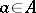. Forone arrives at the definition of an endomorphism. All endomorphisms of a polygonform a monoid, andcan be considered as a polygon over it.

An equivalenceon an-polygonis called a congruence if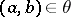implies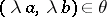for any. The set of congruence classes ofis naturally transformed into an-polygon, called a quotient polygon of the polygonand denoted by. Ifis a polygon over, then inone can define a relation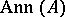by putting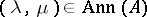if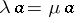for all. The relationis a congruence on the monoid, andis transformed in a natural fashion into a polygon over the quotient monoid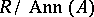. If the polygonarose from a certain automaton, then this transition is equivalent to identifying identically acting sequences of input signals. In universal algebra one considers the usual constructions of direct and subdirect product, but in addition in polygon theory one may consider a wreath product construction important in the algebraic theory of automata. The free product (or co-product) of polygons coincides with their disjoint union.

A polygon may be regarded as a non-additive analogue of a module over a ring, which serves as a rich source of problems in the theory of polygons. In particular, a relationship has been established between polygons and radicals in semi-groups (cf. Radical in a class of semi-groups), and studies have been made on the relation between the properties of a monoid and those of polygons over them. For example, all left-polygons are projective if and only ifis a one-element group, while the injectivity of all polygons over a commutative monoidis equivalent to the presence inof a zero and the generation of all its ideals by idempotents (see Homological classification of rings).

Ifis a monoid with zero 0, one can speak of an-polygonwith a zero as an-polygon with a distinguished pointsatisfying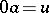for all. The theory of polygons containing zeros has some special features.

Every polygon can be considered as a functor from a one-object category into the category of sets.

## Contents

How to Cite This Entry:
Polygon (over a monoid). Encyclopedia of Mathematics. URL: http://encyclopediaofmath.org/index.php?title=Polygon_(over_a_monoid)&oldid=36988
This article was adapted from an original article by L.A. Skornyakov (originator), which appeared in Encyclopedia of Mathematics - ISBN 1402006098. See original article